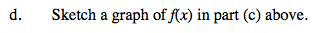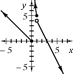### Home > CALC > Chapter 1 > Lesson 1.2.1 > Problem1-21

1-21.
1. Given the following functions, compute the given values. Homework Help ✎

1.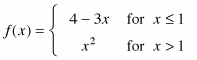Find f (0), f (l),and f (3).

2.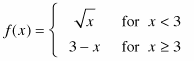Find f (l), f (3),and f (9.4).

3.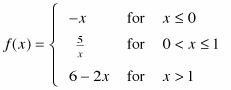Find f (−3), f (0), f (0.5) and f (4).

4. Sketch a graph of f(x) in part (c) above.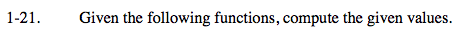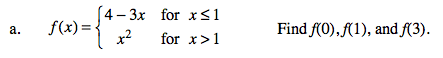x = 1 can be called, informally, the Boundary Point of the piecewise function f(x). This is because x ≤ 1 is the location where the left and the right pieces switch.

f(0) and f(1) are on the left side of the Boundary Point, f(3) is on the right side of the Boundary Point.

f(0) = 4 − 3(0) = 4 LEFT PIECE
f(1) = 4 − 3(1) = 1 LEFT PIECE
f(3) = (3)² = 9 RIGHT PIECE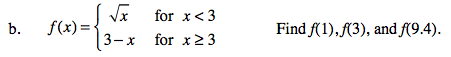The Boundary Point is x ≥ 3. So f(1) lies on the left side of the piecewise function while f(3) and f(9.4) lie on the right.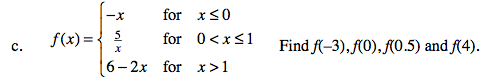This function has two boundary points and three pieces: a left piece, a middle piece and a right piece. On which piece do the points f(−3), ff(0), f(0.5) and f(4) lie?